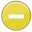#normality 中文解釋 wordnet sense Collocation Usage Collins Definition
Noun
/nôrˈmalitē/,Font size:The condition of being normal; the state of being usual, typical, or expected,
1. The condition of being normal; the state of being usual, typical, or expected
• - let's try to get some normality back in our lives

1. being within certain limits that define the range of normal functioning
2. (of a solution) concentration expressed in gram equivalents of solute per liter
3. expectedness as a consequence of being usual or regular or common
4. conformity with the norm
5. In behavior, normal refers to a lack of significant deviation from the average. The phrase "not normal" is often applied in a negative sense (asserting that someone or some situation is improper, sick, etc.) Abnormality varies greatly in how pleasant or unpleasant this is for other people.
6. In category theory and its applications to mathematics, a normal monomorphism or conormal epimorphism is a particularly well-behaved type of morphism. A normal category is a category in which every monomorphism is normal. A conormal category is one in which every epimorphism is conormal.
7. In chemistry, concentration is the measure of how much of a given substance there is mixed with another substance. This can apply to any sort of chemical mixture, but most frequently the concept is limited to homogeneous solutions, where it refers to the amount of solute in the solvent.
8. \; e^{ -\frac{(x-\mu)^2}{2\sigma^2} } | cdf = | mean = μ | median = μ | mode = μ | variance = σ2 | skewness = 0 | kurtosis = 0 | entropy = | mgf = | char = | fisher = }}
9. Normality is a 3D graphical adventure game, released in June 1996 by Gremlin Interactive. All the cut-scenes in this game are pre-rendered in fact it was one of the first few games out there, which uses exclusievely pre-renderend graphics. ...
10. A measure of substance equivalents that are dissolved in a volume of solution. Equivalents are a measure of the actual mass of material divided by the equivalent mass. That equivalent mass is the atomic mass of the compound divided by the valence of the compound. ...
11. is a concept related to molarity, usually applied to acid-base solutions and reactions. For acid-base reactions, the equivalent is the mass of acid or base that can accept or donate exactly one mole of protons (H^+ ions). Normality is also used for redox reactions. ...
12. The psychological characteristics attributed to the majority of people in a population at a given time.
13. Show an "N" on the DH and place the NDH [bC], PO > DS, FO up, next to the letter with pc. (< uppercase "N" used as an abbreviation for "normality")